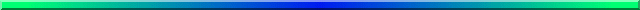# Multiple Imputation Using Amelia

## David C. Howell# Using Amelia to Handle Missing Data

I want to state right at the beginning, I am not an authority on Amelia. In fact, I'm a newby. So this page will be very basic, but I think that it is accurate. I have put it together because Amelia is quite a nice, though old, package. You may have better luck with other programs about which I have written pages.

Matt Owen , Kosuke Imai , Gary King , Olivia Lau wrote Amelia. Amelia is a program that will take your raw data, with missing data, and create multiple data files that have the missing data filled in. It is quite easy to set up. The following code will do the first part.

```	# Barebones code for Amelia

# Amelia does the multile imputations, but you need the  Zelig package to complete the analysis.
# We will do the analysis on the multiple imputations and then combine.
# The following is very much a barebones approach. Sorry.
# Good sources are
# http://cran.r-project.org/web/packages/Amelia/vignettes/amelia.pdf
# https://lists.gking.harvard.edu/pipermail/amelia/2015-January/001125.html

library(Amelia)
### First set working directory
attach(data)    # I know--it is a terrible thing to use attach()
imputations <- amelia(data, m = 5, p2s = 1)
save(imputations, file = "AmeliaImps.RData")
write.amelia(obj = imputations, file.stem = "AmeliaImps")
# The first saves it to a system file, and the second writes to a text file
### The following just does the regression on the first set of imputed data  JUST A DEMO
imp1run <- lm(totbpt ~ sexp + deptp + anxtp + depts + anxts  , data = out\$imputations\$imp3)
summary(imp1run)
```

The output, not shown, will show you how quickly the solution stabilized for each set of imputations, and then would then show the multiple regression using the first set of imputations.

But now that you have the data sets, you need to combine them in some way. A library called "Zelig" will do that for you, but it takes a bit of hunting to figure out just how to do that. See the references I gave above.

The necessary code using Zelig is shown below.The first set of printout shows you the combined result using Rubin's rules discussed elsewhere. These are not a terrific match with results you have seen elsewhere, but they are what we have.

```###       R CODE
# Now to combine the sets of imputations
install.packages("Zelig")
library(Zelig)
z.out<- zelig(totbpt ~ sexp + deptp + anxtp + depts + anxts, data = imputations, model = "ls")
print(summary(z.out))
# If you read the bottom of that printout you come up with the following. They look almost
# identical, but notice that the second puts z.out in parentheses.
summary(z.out, subset = 1:5)           # Generates the combined results
print(summary(z.out))

#       OUTPUT-part 1
Model: ls
Number of multiply imputed data sets: 5

Combined results:

Call:
lm(formula = formula, weights = weights, model = F, data = data)

Coefficients:
Value        Std. Error      t-stat         p-value
(Intercept)   -8.0055490    7.6899690     -1.0410379      3.064899e-01
sexp          -2.8789677    2.6250305     -1.0967368      2.946007e-01
deptp         0.8979177     0.2132500      4.2106351      5.490913e-03
anxtp        -0.1020967     0.1900241     -0.5372829      6.074100e-01
depts        -0.3782091     0.1173556     -3.2227601      2.498085e-03
anxts         0.7269407     0.1224058      5.9387783      1.122492e-05

For combined results from datasets i to j, use summary(x, subset = i:j).
For separate results, use print(summary(x), subset = i:j).

#        OUTPUT - part 2
#  I am only showing the first 2 parts, but you can guess what the next three would look like.

> print(summary(z.out), subset = 1:5)    # Print results for eah imputation

Model: ls
Number of multiply imputed data sets: 5

Result with dataset 1

Call:
lm(formula = formula, weights = weights, model = F, data = data)

Residuals:
Min       1Q   Median       3Q      Max
-15.2326  -4.9405   0.2115   4.8614  14.2993

Coefficients:
Estimate    ß Std. Error   t value     Pr(>|t|)
(Intercept)   -12.1432     6.4620     -1.879       0.06373 .
sexp               -5.1171     1.8505      -2.765       0.00701 **
deptp              1.1165     0.1025    10.896       le 2e-16 ***
anxtp              -0.3442    0.1079     -3.191       0.00200 **
depts              -0.3462     0.1166     -2.970       0.00389 **
anxts               0.8577     0.1023      8.388       1.1e-12 ***
---
Signif. codes:  0 ‘***’ 0.001 ‘**’ 0.01 ‘*’ 0.05 ‘.’ 0.1 ‘ ’ 1

Residual standard error: 6.66 on 83 degrees of freedom
Multiple R-squared:  0.7778,	Adjusted R-squared:  0.7644
F-statistic:  58.1 on 5 and 83 DF,  p-value: < 2.2e-16

Result with dataset 2

Call:
lm(formula = formula, weights = weights, model = F, data = data)

Residuals:
Min       1Q   Median       3Q      Max
-12.9335  -2.8692   0.3288   3.3194   9.1631

Coefficients:
Estimate Std. Error t value Pr(>|t|)
(Intercept) -5.94504    4.97803  -1.194   0.2358
sexp        -3.44021    1.36509  -2.520   0.0136 *
deptp        0.83261    0.07284  11.431  le 2e-16 ***
anxtp       -0.03693    0.07551  -0.489   0.6261
depts       -0.35933    0.08250  -4.355 3.77e-05 ***
anxts        0.69852    0.07492   9.324 1.47e-14 ***
---
Signif. codes:  0 ‘***’ 0.001 ‘**’ 0.01 ‘*’ 0.05 ‘.’ 0.1 ‘ ’ 1

Residual standard error: 4.768 on 83 degrees of freedom
Multiple R-squared:  0.8186,	Adjusted R-squared:  0.8077
F-statistic: 74.93 on 5 and 83 DF,  p-value: < 2.2e-16

```

That is pretty brief coverage. If you have something better, or a better example, I would love to see it.dch: